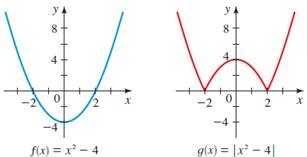# T o explain how the graph g is obtained from the graph of f### Precalculus: Mathematics for Calcu...

6th Edition
Stewart + 5 others
Publisher: Cengage Learning
ISBN: 9780840068071### Precalculus: Mathematics for Calcu...

6th Edition
Stewart + 5 others
Publisher: Cengage Learning
ISBN: 9780840068071

#### Solutions

Chapter 2.5, Problem 85E
To determine

## T o explain how the graph g is obtained from the graph of f

Expert Solution

The graph g , reflect in the xaxis the part of the graph of f that is below the xaxis

### Explanation of Solution

Given information : The graph of f(x)=x24 and g(x)=|x24| are shown belowCalculation : The general rule for graphing the function g(x)=|f(x)| is to reflect all the negative values of the function f through the xaxis

Indeed as shown in the figure the graph of g is obtained if the negative part of f is reflected above the xaxis

That means,

f(x)=x24g(x)=|x24|g(x)={x24;x240(x24);x24<0g(x)={f(x);x240f(x);x24<0g(x)={f(x);x20f(x);x2<4g(x)={f(x)    ; x2f(x) ; -2<x<2f(x)    ; x2

### Have a homework question?

Subscribe to bartleby learn! Ask subject matter experts 30 homework questions each month. Plus, you’ll have access to millions of step-by-step textbook answers!